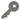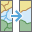# Dissolve BoundariesAvailable in big data analytics.

The Dissolve Boundaries toolaggregates features based on specified attributes. One or more fields can be selected to merge areas with matching attribute values.

## Example

An agricultural development company sampled thousands of terrestrial quadrats across a county. The data includes a soil_depth field and a suitability field. The values in the suitability field were calculated by classifying soil_depth values into categories of low or high. The quadrats can be dissolved based on the suitability field to determine where the most expansive suitable area is located.

## Usage notes

• The input must be a single layer of polygon features. The dissolved output features will always be polygon features.
• You can also calculate statistics on numerical fields for areas that are being dissolved together.
• Output can include multipart or single-part features. A multipart feature is defined as one feature that is divided into noncontiguous parts. For example, the state of Hawaii can be considered a multipart feature because its separate geometric parts are classified as a single state.
• If no fields are specified for the Dissolve Fields parameter and Multipart is set to Yes, the output will be one multipart feature. If no fields are specified for the Dissolve Fields parameter and Multi-part is set to No, only polygons that intersect will be merged.
• Additional statistics can be calculated using the Summary Fields parameter. The summary fields statistics available depend on the field type you are summarizing. A string attribute field can use the Any, Count, and Count Distinct statistics. A numeric attribute field can use the Any, Count, Count Distinct, Sum, Sum of Squares, Min, Max, Range, Variance, and Standard Deviation statistics. A date attribute field can use the Any, Count, Min, Max, and Range statistics.

## Parameters

ParameterDescriptionData type

Input Layer

The point features for which density will be calculated.

Features

Dissolve Fields (optional)

The field or fields for which features will be aggregated. Features with the same field values will be dissolved.

String

Summary Fields (optional)

The statistics that will be calculated for specified fields. Different statistics are available depending on whether the specified field is a string, numeric, or date field.

• Any—This is a sample string from a field of type string.
• Count—Calculates the number of nonnull values. It can be used on numeric fields or strings. The count of [null, 0, 2] is 2.
• Count Distinct—Calculates the number of distinct, nonnull values. It can be used on numeric fields or strings. The count distinct result of [null, 4, 3, 4] is 2.
• Sum—The sum of numeric values in a field. The sum of [null, 1, 3] is 4.
• Sum of Squares—The sum, over all observations, of the squared differences of each observation from the overall mean. The sum of squares of [null, 2.2, 3.1, 4.7] is 3.206.
• Min—The minimum value of a numeric field. The minimum of [0, 2, null] is 0.
• Max—The maximum value of a numeric field. The maximum value of [0, 2, null] is 2.
• Mean—The mean of numeric values. The mean of [0,2, null] is 1.
• Range—The range of a numeric field. This is calculated as the minimum value subtracted from the maximum value. The range of [0, null, 1] is 1. The range of [null, 4] is 0.
• Variance—The variance of a numeric field in a track. The variance of  is null. The variance of [null, 1,1,1] is 1.
• Standard Deviation—The standard deviation of a numeric field. The standard deviation of  is null. The standard deviation of [null, 1,1,1] is 1.

String

Multipart

Specifies whether multipart features are allowed in the output layer. A multipart feature is defined as one feature that is divided into noncontiguous parts.

• Yes—The output will create multipart features if applicable.
• No—Only single-part features will be created in the output.

Boolean

## Output layer

The output layer will contain the following:

• Fields specified in the Dissolve Fields parameter.
• A COUNT field that represents the number of features that were dissolved into the feature.
• Summary field values configured for the tool.

## Considerations and limitations

The Dissolve Boundaries tool can only be used on polygon features.